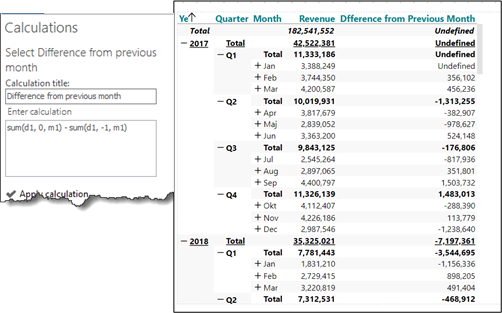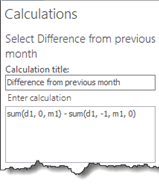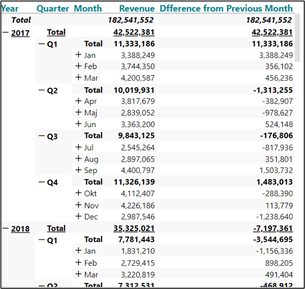# The 4th parameter

The 4th parameter is useful when referencing out of range in relation to the dataset that has been defined for the crosstab at hand.

This will normally result in the error message “not defined”. By using the 4th parameter you can insert a numeric value which will replace the error message.

An example:

• Create a new analysis Monthdifference with a crosstab showing Revenue per Time Hierarchy (Month).
• Add a calculation showing the difference between current month and the previous month.

The formula could be: sum(d1, 0, m1) – sum(d1, -1, m1)

In this particular case this formular will return “Undefined” in the first row – because there is no previous month in the dataset – and the reference is out of range.In this case the 4th parameter can be a solution.

New formula: sum(d1, 0, m1) – sum(d1, -1, m1, 0)

The last 0 indicates that the value 0 should be inserted in case of reference out of range.

Now the first row (January) is calculated as if the previous month was 0.